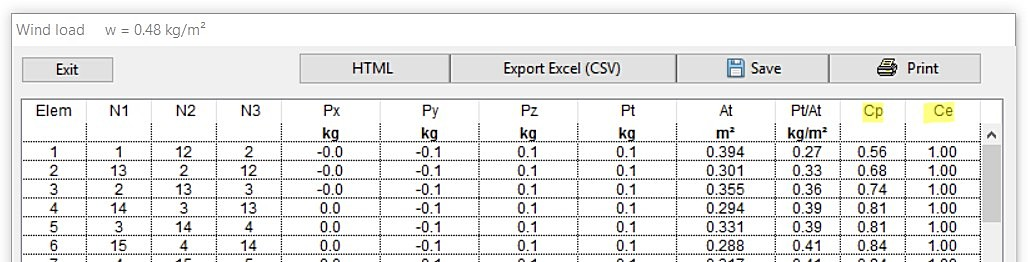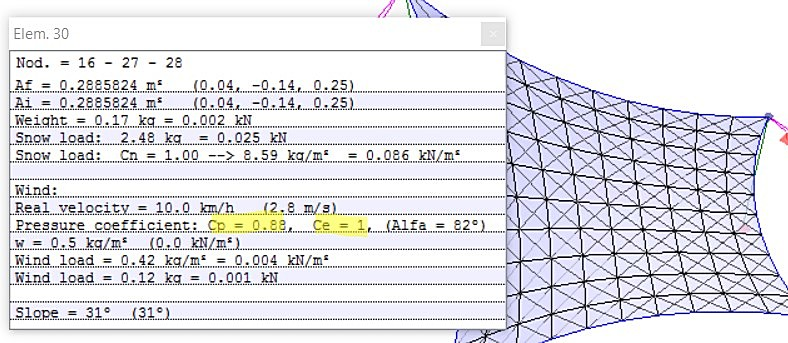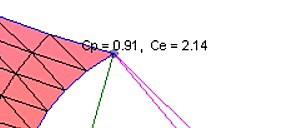##### Preamble

WinTess is designed to be used anywhere in the world. Each country has its own standard of construction, therefore, unless all these rules are introduced into the program, it is impossible to meet each user’s own standards. In this sense WinTess facilitates the possibility to manually modify the calculation of wind actions in order to meet each local standard.

##### Introduction

Wind actions in WinTess are treated as uniform loads for each individual surface element (triangle). Each element has a surface area At and a normal N. “normal” is a vector perpendicular to the surface element pointed out. Outside means as the side of blue color in the software.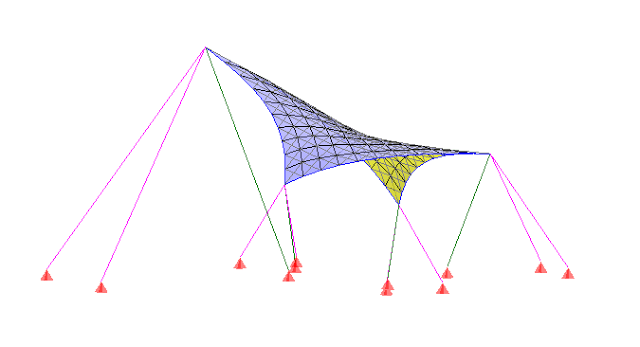The uniform wind wl in each element is:

`wl = w · Cp · Ce`

where is w the dynamic pressure of the wind. This is a value known in fluid mechanics and acceptable to wind

`w = v² / 1.6`

where v is the speed of wind in (m/s) and w (N/m²)

So for a wind of v = 100 km/h = 27.8 m/s, we obtain the value of w = 0.482 kN/m²

Cp (Pressure Coefficient) is a value that depends on the direction of the normal N relative to the direction of the wind and the location of the element in the membrane (Center, edge,…)

Ce (Exposure Factor) is a value that modifies the speed of the wind, according to the type of terrain where the membrane is located and height relative to the ground level.

##### Wind speed

WinTess allows user to enter wind speed in two different ways: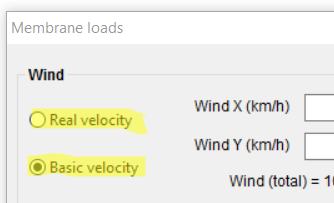Real Velocity

This option is useful when we want to adapt to local legislation which is not approaching the way of applying the action of the wind according to WinTess. It is necessary to get this value from the data supplied by a meteorological station or similar, paying special attention to local micro climates, since the wind can change much from one place to another.

Basic Velocity

Most of the current regulations consider basic wind speed as a minimum value. The actual wind speed can be obtained through multiplication factors of Ce, taking into account the type of terrain, the height of the point on the ground, etc. The wind speed should be defined in the correct direction. To facilitate this action WinTess allows you to enter the X and Y components independently. You can also use negative values. WinTess automatically displays the total value of wind speed and the dynamic pressure w.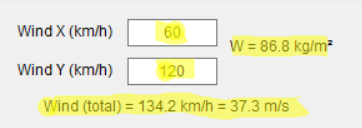Cp Value

To calculate the value of Cp for each surface element WinTess provides three options:

1. Cp manual
This can be done using the menu Calculate | CP manual or by using the corresponding check box in the window for surface loads.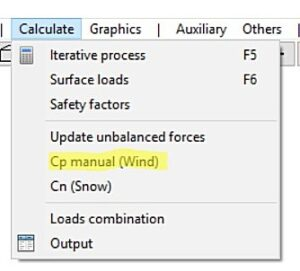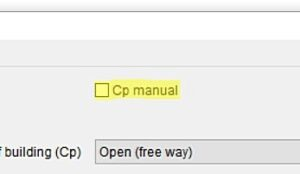If the user chooses this method, you need to select the elements (one by one or grouped in a window) and assign the appropriate value Cp. At any time, user can change any of these settings via the menu Calculate | Cp manual.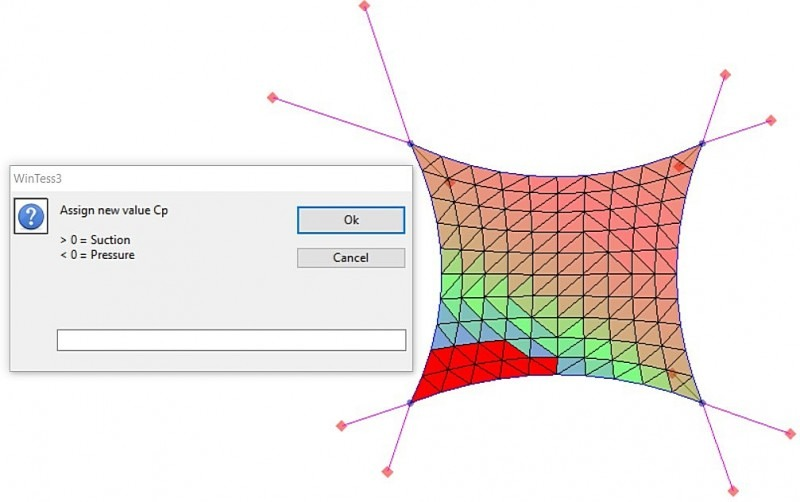2. Type of Building

WinTess provides some models or “types” of building. It is very likely that occasionally other types of building are added. At this time (V 3.136), there are these types: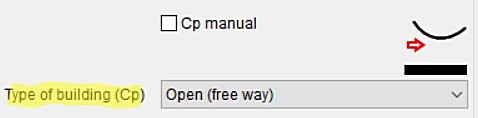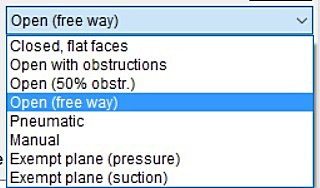Each building type automatically populates the different values in the table of Cp: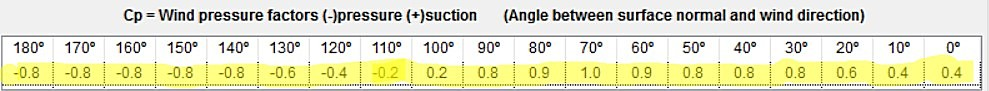Each Cp value in the table corresponds to each angle formed by the direction of the wind vector and the normal N. An angle between two vectors is between 0° (matching) and 180° (opposite). These Cp values are obtained from different sources, so the user should check if these values are appropriate for the case being studied. The values which the user does not like can be modified using the Manual option in the drop down list for Types of building. If you select Manual from this drop down list, the Cp values become editable and can be changed.

3.Border effect

It is well known that due to the turbulence around the edges of the buildings, wind actions should be considered greater in these areas. If the user checked the Border effect box, WinTess automatically modifies values of Cp of the elements that have one side coinciding with the perimeter of the membrane.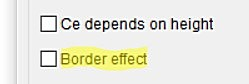In fact the Cp value will be multiplied by 1.5. It’s a quick approximation, therefore if this approach is not liked, the user must manually modify these values by using the option Cp manual introduced earlier. In this way you can modify not only those which directly touch the edge, other elements as well.

4.Ce Value

WinTess automatically calculates the Ce value for each element. If real velocity is used, then always Ce = 1. If you use basic velocity, then Ce depends on:

Height above the ground (Ze)

If we check the Ce box depends on height, WinTess take Ze as the Z-coordinate of the center of gravity of the element in question. If we do not check it, all elements use the same Ze. This value is then calculated as the weighted average of the Z-coordinate of the center of gravity of each of the elements that make up the membrane. In any case, it is very important to locate the origin of coordinates in such way that ground corresponds to a value Z = 0.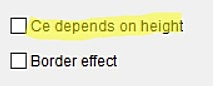Terrain Type

Depending on the type of chosen terrain, Ce will take a particular value. First, we will define: k, L and Z

```Sea or coastal area                   k = 0.156 L = 0.003 Z = 1
Flat area without obstacles           k = 0.17  L = 0.01  Z = 1
Low vegetation and isolated obstacles k = 0.19  L = 0.05  Z = 2
Forests and urban areas               k = 0.22  L = 0.3   Z = 5
Among high buildings                  k = 0.24  L = 1     Z = 10```

We then define F and Ce

```F = k * ln(Max(Z, Ze) / L)
Ce = F * (F + 7 * k)```

Checking Cp and Ce

At any time we can check the values of Cp or Ce for each element. You have three possibilities: